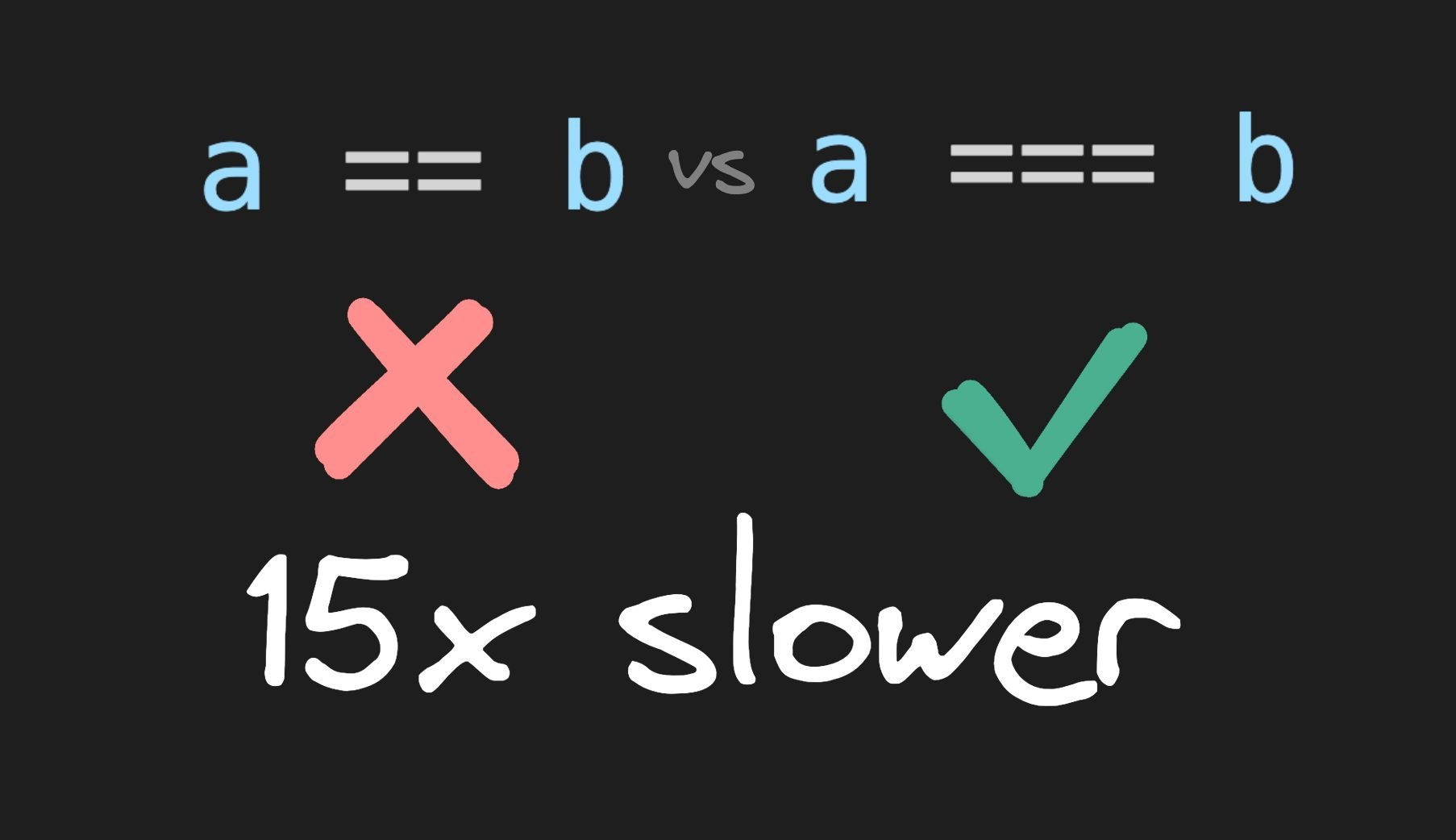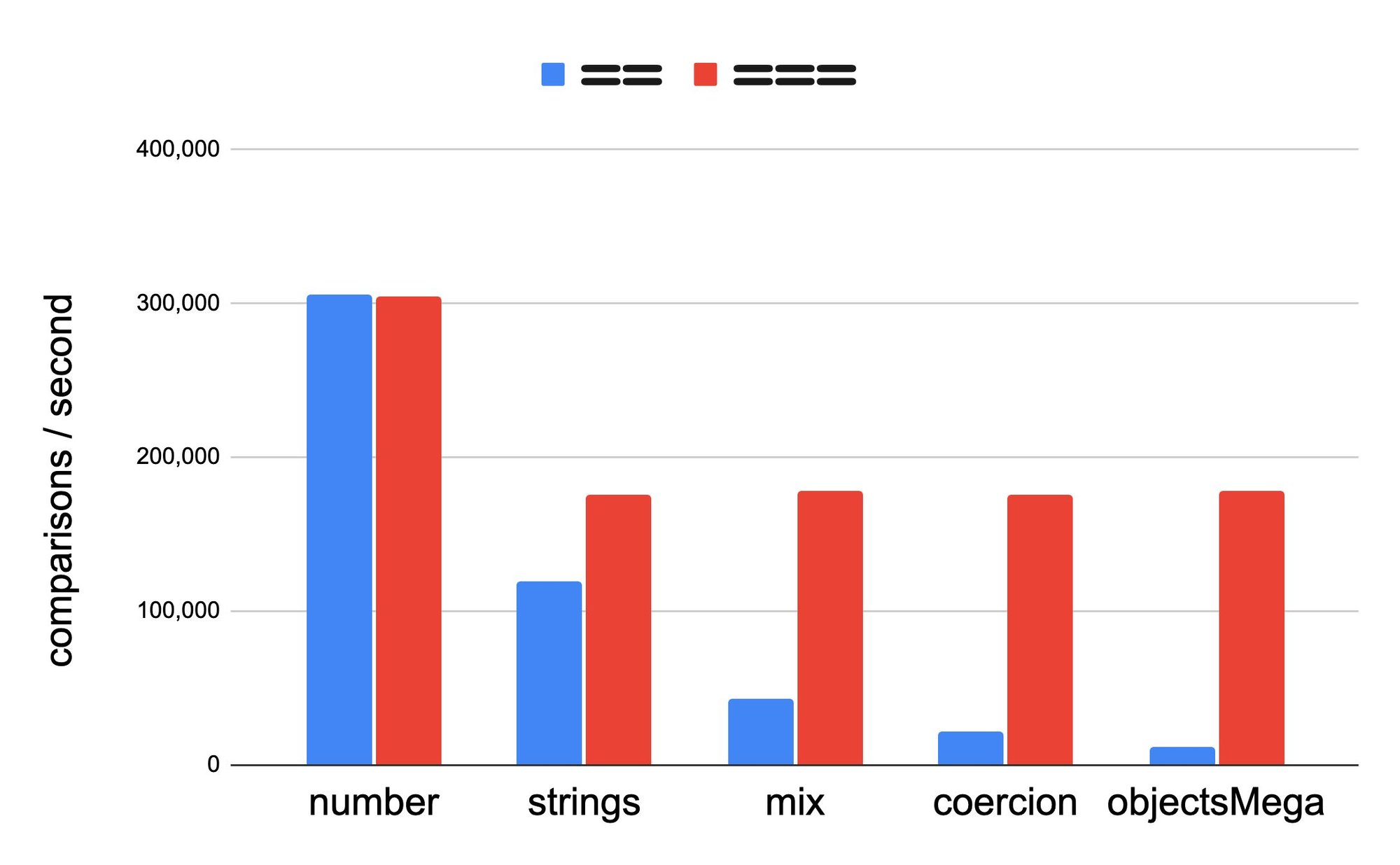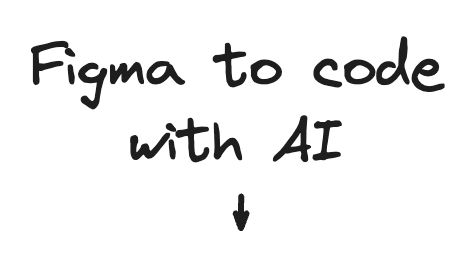Visual Copilot Livestream | Dec 6 @10am PSTIntroducing Visual Copilot: Figma to code with AITalk to Us
Product
Solutions
Developers
Talk to UsBlog

Home

Resources

Blog

Forum

Github

Signup

×

Visual CMS

Drag-and-drop visual editor and headless CMS for any tech stack

Theme Studio for Shopify

Build and optimize your Shopify-hosted storefront, no coding required

Resources

Blog‹ Back to blog

# How can == be up to 15 times slower than ===?

#### Written ByMiško HeveryWe all know that JavaScript has both `==` (equality) and `===` (strict equality) operators for comparison. But what exactly is the difference, and more importantly, what is happening under the hood? Let's dive in!

## The difference

The `==` is a coercion comparison. Here, coercion means that the VM tries to force the two sides to the same type and then see if they are equal. Here are a few examples of things that are automatically forced into equality:

``````"1" == 1 // true
1 == "1" // true
true == 1 // true
1 == true // true
 == 1 // true
1 ==  // true``````

The coercion is symmetric, if a == b is true, then b == a is true as well. The ===, on the other hand, is true only if the two operands are precisely the same (except for Number.NaN). So, none of the above examples will be true with ===.

The actual rules are complicated (which is a reason in itself to not use `==`). But to show just how complicated the rules are, I have implemented the `==` using the `===` only.

``````function doubleEqual(a, b) {
if (typeof a === typeof b) return a === b;
if (wantsCoercion(a) && isCoercable(b)) {
b = b.valueOf();
} else if (wantsCoercion(b) && isCoercable(a)) {
const temp = a.valueOf();
a = b;
b = temp;
}
if (a === b) return true;
switch (typeof a) {
case "string":
if (b === true) return a === "1" || a === 1;
if (b === false) return a === "0" || a === 0 || a == "";
if (a === "" && b === 0) return true;
return a === String(b);
case "boolean":
if (a === true) return b === 1 || String(b) === "1";
else return b === false || String(b) === "0" || String(b) === "";
case "number":
if (a === 0 && b === false) return true;
if (a === 1 && b === true) return true;
return a === Number(String(b));
case "undefined":
return b === undefined || b === null;
case "object":
if (a === null) return b === null || b === undefined;
default:
return false;
}
}

function wantsCoercion(value) {
const type = typeof value;
return type === "string" || type === "number" || type === "boolean";
}

function isCoercable(value) {
return value !== null && typeof value == "object";
}
``````

Wow, that is complicated, and I am not even sure it is correct! Maybe someone else knows of a simpler algorithm, but this is the best I could do. See implementation.

It is interesting to note that if one of the operands is an object, the VM invokes `.valueOf()` to allow the object to coerce itself into primitive types.

OK, that implementation is complicated. So how much more expensive is `==` than `===`? Check out this chart. (See benchmarks here.)Higher is faster (more operations per second.)

First let's talk about number arrays. When a VM notices that the array is pure integers, it stores them in a special array known as `PACKED_SMI_ELEMENTS`. In that case, the VM knows that it is safe to treat `==` as `===` and the performance is the same. This explains why there is no difference between the `==` and `===` in the case of numbers. But as soon as the array contains things other than numbers, things start to become bleak for `==`.

With `strings`, there is a 50% decrease in performance of `==` over `===` and it only gets worse from there.

Strings are special in VM, but as soon as we get objects involved, we are 4x slower. Look at the `mix` column the slowdown is now 4x slower!

But it gets even worse. An object can define `valueOf` in order to coerce itself into a primitive. So now the VM has to call that method. Of course, locating properties on objects is subject to inline caching. Inline caching is the fast path for property-read, but a megamorphic read can experience a 60x slowdown, which can make the situation even worse. As shown in the graph as a worst-case (`objectsMega`) scenario, the `==` is 15 times slower than `===` ! WOW!

Now, `===` is very fast! So even a 15x slowdown with `===` is not something that will make much difference in most applications. Nevertheless, I am struggling to think of any reason why one should use `==` over `===`. The coercion rules are complex, and it is a performance footgun, so do yourself a favor and think twice before using `==`.

Builder.io is a visual editor that connects to any site or app and lets you drag and drop with your components.

Try it out
``````// Dynamically render your components
export function MyPage({ json }) {
return <BuilderComponent content={json} />
}

registerComponents([MyHero, MyProducts])``````Introducing Visual Copilot: a new AI model to convert Figma designs to high quality code in a click.

No setup needed. 100% free. Supports all popular frameworks.

Try Visual Copilot#### ShareIntroducing Visual Copilot:

A new AI model to turn Figma designs to high quality code.

Try Visual CopilotLike our content?